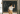# Why Is the Square Root of 25 Equal to 5? Time To Understand Principal Square Roots!

POSTED ON APRIL 05, 2023When dealing with principal square roots, the focus is on the positive square root of a number. In this article, learn how to identify the principal square roots and understand why the principal square root of 25 is 5!

## What Is a Principal Square Root? How Does It Apply to the Square Root of 25?

The principal square root refers to the positive square root of a number. Recall that taking the square root is simply the process of reversing the process of squaring a number. Every nonnegative number has two square roots: the positive and negative roots. When dealing with the principal square root, focus on the positive root alone.To better understand the concept of square roots and principal square roots, take a look at the square root of 25. 25 is a perfect square and is the result of squaring either -5 or 5.Online Tutoring with Juni Tutor Bot - Get Free Help 24/7
Juni Tutor Bot is your friendly online tutoring assistant. Get immediate, free homework help and exam support for general math, calculus, algebra tutoring, and standardized test prep like SATs. Learn with a community of peers and tutors, supported by AI.

Juni Learning has excellent resources that will help you in establishing strong foundations in algebra. Discover the different techniques and methods offered by Juni Learning’s materials to support you in mastering Common Core topics. Juni Learning will not only help you with the basics, but it can be your overall ally when it comes to learning advanced topics for math and other subjects!

The square root of 25 is equal to -5 and 5, so the principal square root of 25 is equal to 25. The concept of principal square root applies to both a perfect square and a nonperfect square number - as long as they’re real and nonnegative. Here are examples of numbers with their square roots and principal square root:You can see that whether you’re working with perfect squares and nonperfect squares, there will always be one principal square root.